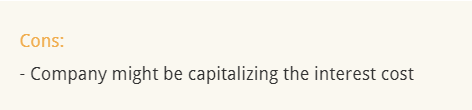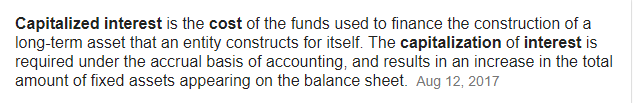# Interest cost capitalization?what does this meanAssume you borrow 1000 from a bank @ 12%. The cost of funds is 120 per year. Further assume I’ve borrowed this money to construct a building over 2 years, after which I intend to repay the principle of Rs.1000.

Now, assume the following is P&L statement of the company.

Year 1 -
Revenue Generated = 2000
Expenses = 1500
Finance charge/Interest Cost = 60
Profit for the year = 440

Now, you’d ask me why have I shown only 60 as finance charge when I actually repaid 120 as interest cost. This is because, out of Rs.1000, I borrowed, I utilized only 500 towards the building, on which the interest applicable is 60. So although I paid 120, I will show the interest cost only to the extent of which I utilized the funds. Companies tend to do this to show a better profit after tax.

This is essentially, capitalization of interest. So whenever you see the interest charges, make sure you check the associated notes to figure out if the company is actually capitalized the interest.

Also, do note, capitalizing interest is perfectly valid accounting technique.

3 Likes